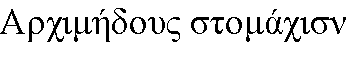The first chapter is devoted to one of the great ancient minds, whose ideas still impact our time. He lived 287 - 212 BC and his life ended tragically through senseless military violence. His countless mathematical insights and concepts cannot be shown here, but the Stomachion attracted me and I spend some time with deep investigation.

The Stomachion is the oldest known puzzle and with an age of over 2000 years the great-grandfather of all puzzles. It consists of a number of figures that you can arrange in a square of 12 by 12 units. The Stomachion has 536 different solutions, if you discard symmetric solutions. I was surprised that this result is despite of its age only recently confirmed by a computer program. (see e.g. [Wolfram] Stomachion).

The solution can easily be calculated with the Logelium, if we take some additional ideas into consideration. The depth of the search tree is relatively small, so all solutions are found quickly. Even my lame laptop takes only a few seconds.

That does not at all mean that the Stomachion is a trivial problem. It contains on the contrary plenty of unexplored or unnoticed properties. Maybe I only found for myself what has been known already for a long time, but the fun is the same.To simplify the Archimedes Stomachion we at first have to understand that both parts of tile A must always come paired. The reason is that the smaller triangle has a side ratio of 2:3 an therefore contains the irrational angle arctan(2/3) = 33°.69006… . But most of the tiles contain the angle arctan(½) = 26°.56505… , which appears between the base line and the diagonal line of two squares. This angle is denoted »λ« in the following. Because the sum of all angles at vertex points is 360° naturally, this is only possible with compensating λ angles. The other irrational angles never add up to an integer result. Similar considerations work for tile B and C, which contain the angle arctan(¼) = 14°.03624… .

Now we can safely merge both parts of tile A and B without impact on the number of solutions. If we do the same with tile C, we find that this shape now occurs two times. This results in dividing the number of solutions by two, if we define both C tiles as equal. There a consequently 268 solutions (PDF) of the simplified Stomachion. You can easily construct the full solution set by cutting one of the C tiles in each of the solution, if you like.

### Description

A
24+3
90°, 90°, 90°+λ, 90°-λ
both parts of the tile only fit the way shown
B
12+12
λ, 45°, 135°-λ
dito
C
3+6
λ, 90°, 90°-λ
dito, but now we have two C tile with the same outline
D
6
45°-λ, 2*λ, 135°-λ
the only tile with no integer size edge
E
12
λ, 135°, 45°+λ, 180°-2*λ

F
6
λ, 45°, 135°-λ
two times
G
12
90°-λ, 45°, 45°+λ
two times
H
21
90°, 90°, 135°, 180°-λ, 45°+λ
the only pentagon

This picture shows nicely the angles at the connecting corners:All tiles of the simplified Stomachion are combinations of the cells X,Y,Z and Q, where cell Z only occurs at the top of tile D. Each cell fits exactly into the base grid. Therefore all tiles which are combinations of these cells matching on the marked points. The Logelium defines tiles with the help of these cells and uses them for the puzzle configuration.Especially the left picture shows, that all vertex points hit the base grid. This is a property of all solutions, and raised some amazement already. This property is at the same time a precondition for the Logelium algorithm to find all solutions. The reason becomes clearer after understanding kite rings (see [Logelium] Kite Rings).

If we assume solutions with tiles, which have support points not fitting into the base grid, these solutions must contain a complete kite ring made of cells type »X«. Support points are all marked points of the cells Q,X,Y,Z. Because the Stomachion tiles contain no kites by themselves, the kite ring is made of a number of half kite pairs each with two figures. That means each tile which takes part in the ring is positioned either outside or inside completely. Furthermore only areas of 30 or 60 are possible (B+D/F or E+G+D/F or H+C). So we understand that kite rings inside the Stomachion square are impossible. The form is simply to small and there are only few tiles. With a large number of Stomachion tiles kite rings are of course constructible. So rings are not impossible in principle, but for this and all other convex forms shown on the next pages.

Impressum